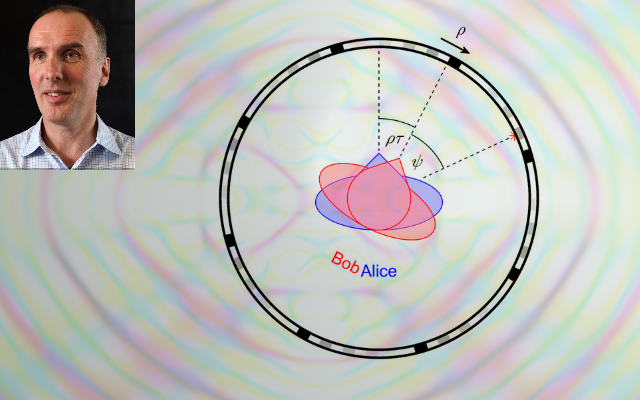# The special relativity of self-rotation perception

A reference frameshift model of spatiotemporal perception, based on the Special Theory of Relativity, explains distortions of perceived simultaneity while experiencing uniform self-rotation, and deviation of default head and eye position while experiencing angular acceleration. A common assumption of neurology is that involuntary eye movements associated with these stimuli (the slow phase of optokinetic and vestibular nystagmus respectively) have an angular velocity predicted exactly to compensate for perceived rotation.  The angular velocity of vestibular nystagmus fits a hyperbolic tangent function of objective uniformly increasing angular velocity. Mathematically, objective is to subjective angular velocity what rapidity is to speed in special relativity. Subjective angular velocity is finite, bounded by 'experiential horizons' of +/- 200deg/s. It is hypothesised that these strange rules of mental representation are an extension to perception of the 'efficient coding hypothesis' of neurophysiology. Here the entropy of the representation (neural or mental) is maximised. Assuming that noise is negligible (the conditional entropy of the representation given the stimulus), this maximises mutual information between stimulus and response. In the case of self-rotation, the hyperbolic tangent objective-to-subjective transfer function suggests that perception would be maximally efficient if objective angular velocities of body rotation over a long period of environmental immersion had a logistic distribution of scale 100 deg/s.
In this talk I will sketch an extension of the efficient perception hypothesis to the geometry of subjective space. I will use this to propose an extension of the self-rotation model to spatio-temporal perception in linear self-motion, both uniform and accelerating. I will outline how a neurologic equivalence principle, between vestibular stimulation and hemispatial neglect, might in the future lead to a mathematical characterisation of attention that is reminiscent of the description of gravity in the General Theory of Relativity.